# AP Physics 1 Multiple-Choice Practice Test 21

### Test Information10 questions18 minutes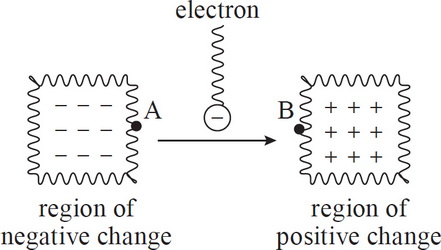1. Which of the following correctly describes an electron moving from point A to point B in the situation above? Assume the two regions of charge are identical in magnitude and only different in sign.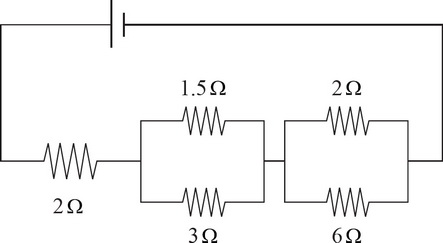2. What is the equivalent resistance of the electric circuit in the picture above?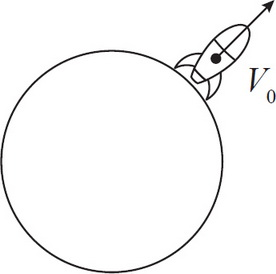3. Escape velocity is defined as the minimum speed at which an object must be launched to "break free" from a massive body's gravitational pull. Which of the following principles could be used to derive this speed for a given planet?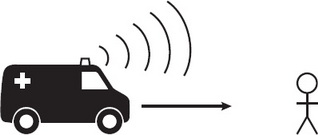4. An ambulance is driving toward you. As it approaches, which of the following correctly describes the changes in the sound of the siren's pitch and intensity?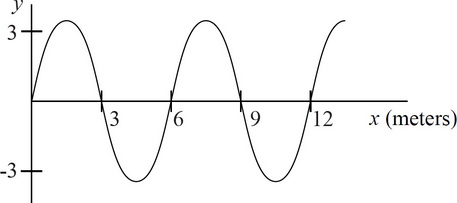5. The graph above depicts a medium's instantaneous displacement from equilibrium, caused by a wave, as a function of distance from the source. If the wave has a speed of 600 m/s, which of the following is the best approximation of the wave's frequency?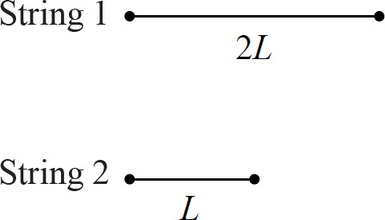6. Both of the above strings have their ends locked in place. The two strings have the same linear density, but the first string, S1, is twice as long as the second string, S2. If sound waves are going to be sent through both, what is the correct ratio of the fundamental frequency of S1 to the fundamental frequency of S2?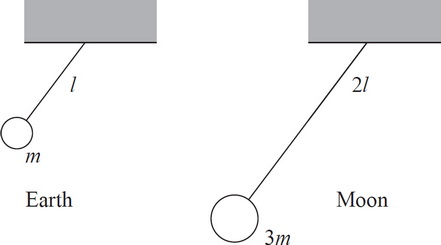7. A pendulum with a ball of mass m hanging from a string of length l is set in motion on Earth, and the system is found to have a frequency of f. If the length of the string were doubled, the hanging mass tripled, and the system moved to the moon, what would be the new frequency?

NOTE: Acceleration due to gravity of the Moon is approximately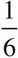of Earth's.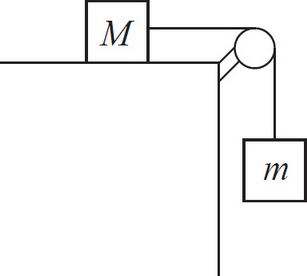8. A block of mass M is at rest on a table. It is connected by a string and pulley system to a block of mass m hanging off the edge of the table. Assume the hanging mass is heavy enough to make the resting block move. Knowing the acceleration of the system and the mass of each block is sufficient to calculate all of the following quantities EXCEPT which one?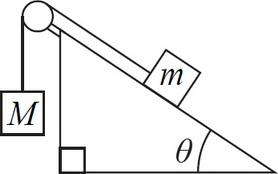9. A block of mass m is connected by a string which runs over a frictionless pulley to a heavier block of mass M. The smaller block rests on an inclined plane of angle θ, and the larger block hangs over the edge, as shown above. In order to prevent the blocks from moving, the coefficient of static friction must be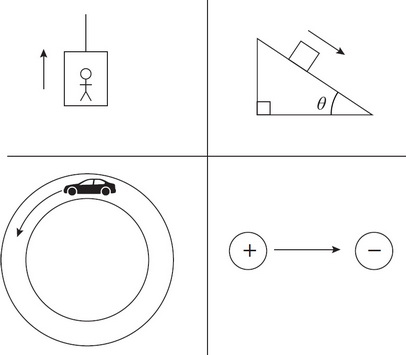10. Which of the following forces does not do work in its given situation?